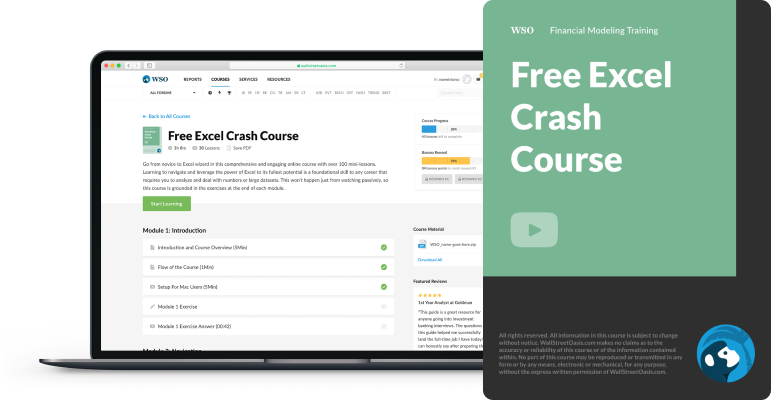# WACC

It is a firm's average cost of capital

The weighted average cost of capital (WACC) is a firm's average cost of capital. It takes into account different types of financing such as common stock, preferred stock, bonds, and other kinds of borrowings.This is a very commonly applied concept in finance. If you're someone who wants to pursue a career in finance, it is essential to understand what the weighted average cost of capital is, when it is used, and how to calculate it. This is also commonly asked about in job interviews.

This is commonly used as the discount rate in discounted cash flow (DCF) analysis to find the net present value (NPV) of an investment. It highlights the time value of money and the risk of holding an investment with uncertain returns.

Companies often use their own weighted average cost of capital as a benchmark against which they measure whether to pursue certain projects or investments. It is the return a company needs to generate to compensate its bondholders and shareholders.

Therefore, it is important that they take it into account as lower returns may make it harder to raise capital to finance future projects.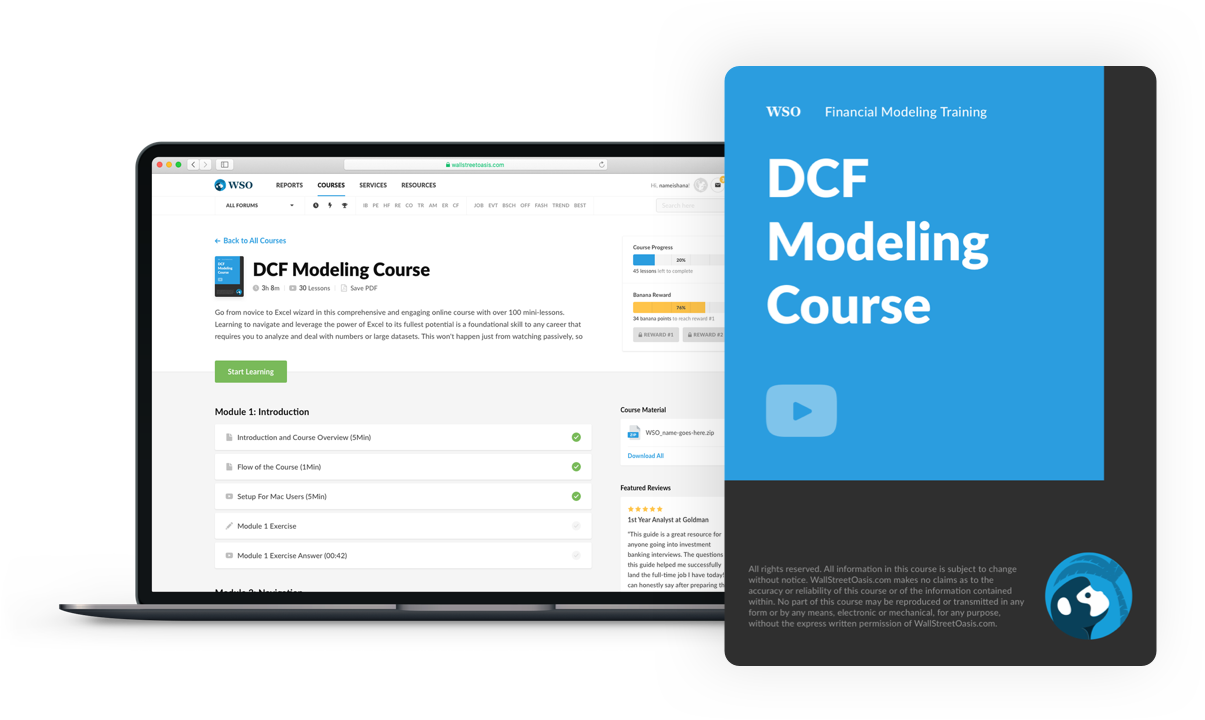### Everything You Need To Master DCF Modeling

To Help You Thrive in the Most Prestigious Jobs on Wall Street.

## How to Calculate WACC?

The weighted average cost of capital is a firm's cost of equity and cost of debt in proportion to their respective share in capital structure. The formula for calculating it is as follows: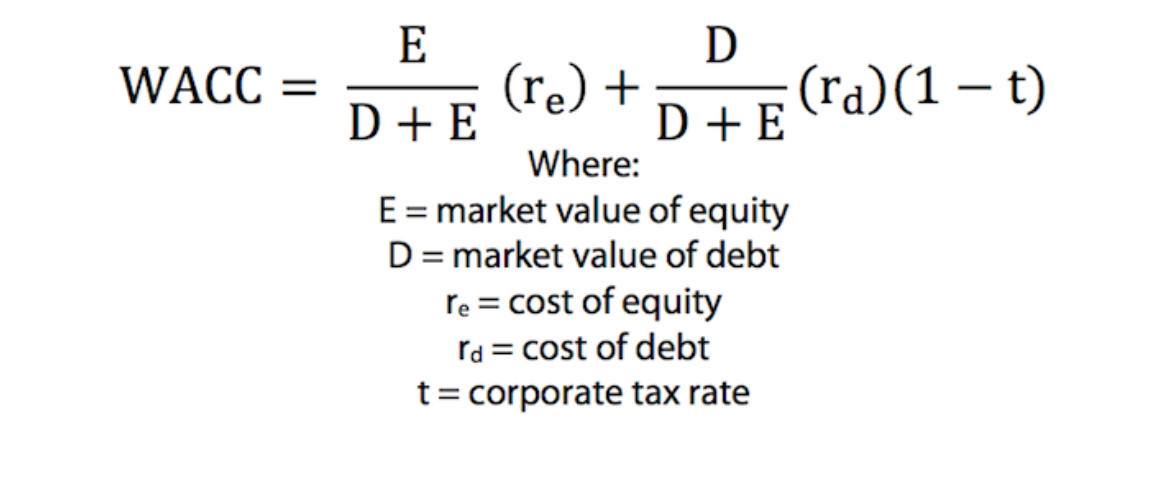E / D + E and D / D + E is the percentage of equity and debt that make up a firm's capital structure.

In this model, the cost of debt is multiplied by (1 - t), which is the tax rate subtracted from one, which lowers the cost of debt in proportion to the taxes the company has to pay. This is because the interest expenses are tax-deductible, and this added value of holding debt is known as a tax shield.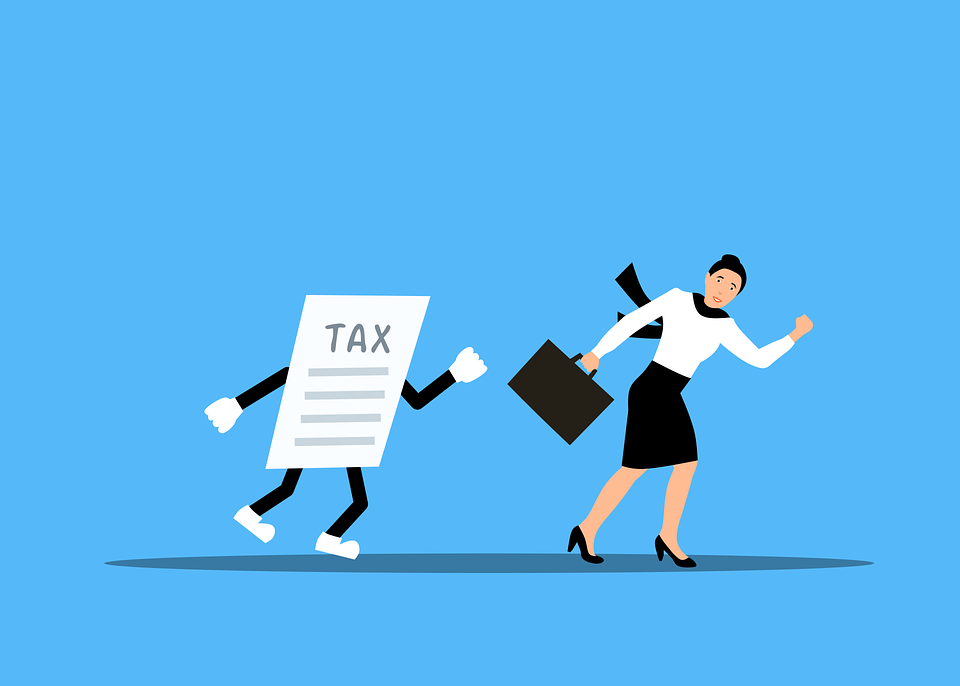### Cost of Equity

To find the weighted average cost of capital, it is necessary to find a company's cost of equity which represents the returns that stockholders expect from their equity interests.

It is based on the risk an investor assumes by holding the company's stock, where the higher the risk, the higher their return expectation (and thereby the cost of equity) and vice versa. Riskiness is usually measured by comparing the volatility of the stock to that of the rest of the market.

The most commonly used measure for the cost of equity is found in the capital asset pricing model (CAPM). The formula is as follows:

CAPM = Rf + (Rm - Rf) * 𝜷

where,

• Rf = Risk-free rate

• Rd = Market return

• 𝜷 = Levered beta

The risk-free rate is the return an investor can expect to make without taking on risk. Usually, investors use the US 10-year treasury bond yield for the risk-free rate.

If investing in foreign assets, it is common to use the 10-year Treasury bond yield of the country where the asset is, as the investment is only as sound as its currency and its government's ability to pay back its debt.

The market return is the expected return of the whole market. It is usually found using historical data to estimate future returns. It is added to the risk-free rate to account for the added risk of holding equities compared to safe assets such as government bonds. This is known as the equity risk premium.A company's beta is its volatility in relation to the whole market. It is found by creating a regression analysis and is the slope of the best fit line. For the cost of equity, levered beta is typically used.

Levered beta is different from unlevered beta because it includes the risk of holding debt in addition to the equity risk. Unlevered beta, on the other hand, assumes that there is no debt in the capital structure.

Levered beta is calculated using the following formula:

Levered Beta = Unlevered Beta * (1 + (1 - Tax Rate) * (Debt / Equity))

As one can see, levered beta is greater than or equal to unlevered beta. This is because a company that holds lots of debt is riskier than a company that is debt free since lenders would be paid before shareholders if it were to go into liquidation.

We use levered beta to measure the cost of equity as the cost of debt is usually less than the cost of equity. Using the levered beta takes into account the added risk associated with holding large amounts of debt that may not be factored into the WACC otherwise.

### Cost of Debt

The cost of debt is much more straightforward than the cost of equity. It is simply the interest that companies pay on their debt.

For the most part, companies take on debt by selling corporate bonds, but they may also have other types of debt, such as bank loans and revolvers.

Usually, they have a mix of short-term and long-term debt in their capital structure, which must be accounted for. Long-term debt is usually more expensive than short-term debt because of the greater uncertainty associated with longer periods of time.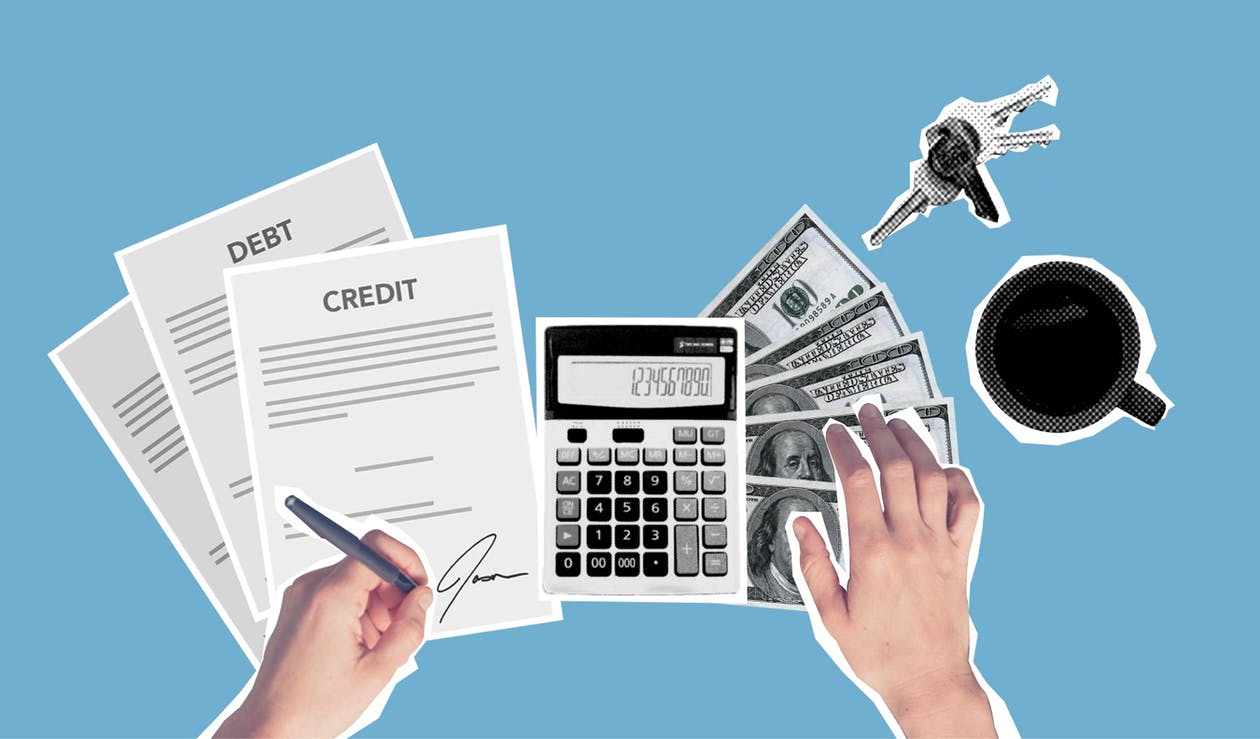### Cost of Preferred Stock

Preferred stock may also be used as a source of financing (is used very sparingly in current times) for companies and should be included in the calculation of the weighted average cost of capital. The cost of preferred stock is calculated by dividing the dividend by its share price.

Cost of Preferred Stock = Preferred Stock Dividend / Price Per Share

This is essentially the yield on preferred stock. Unlike common stock, the dividends on preferred stock are debt obligations that must be paid and are therefore included in the cost of capital.

## Example of WACC

Imagine you're an investor looking to make a potential investment into a company called Sweendog LLC. You understand that risk is involved, but I would like to know what kind of return is fair compensation for the amount of risk you're taking.

It is a good way to measure this. To find a fair return, you need to find the cost of debt, equity, and the capital structure of Sweendog LLC.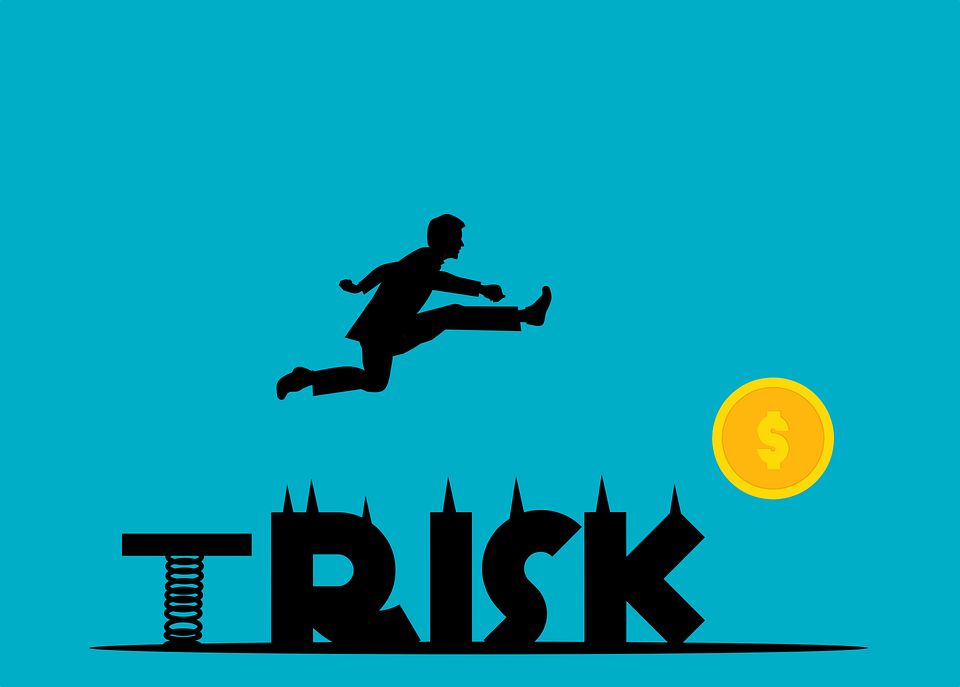Let's find the cost of debt first. Imagine that Sweendog LLC has 70% long-term debt at a 5% yield and 30% short-term debt at a 3% yield. The cost of debt would be calculated as follows:

Cost of Debt = (0.70 * 0.05) + (0.30 * 0.03) = 0.044

This is equal to a 4.4% average yield on debt, which is also the cost of debt.

Next, let's find the cost of equity. Imagine the following:

• The risk-free rate is 2%

• The expected market return is 8%

• Sweendog LLC's leveraged beta is 1.28

The formula to find the cost of equity would be:

Cost of Equity = 0.02 + (0.08 - 0.02) * 1.28 = 0.0968

The cost of equity for Sweendog LLC is, therefore, 9.68%.

Now imagine the company has \$200k in debt and \$800k in equity. To find the weighted average cost of capital, put the cost of debt and cost of equity together in the formula presented earlier!

WACC = (800k / (800k + 200k))(0.0968) + (200k / (800k + 200k))(0.044) = 0.08624

This equals 8.624%. A WACC of 8.624% means that you should be reasonably sure that you will make an 8.634% return on the investment, or else you should consider not investing, as the payoff is not worth the risk.## Limitations of WACC

It is a very commonly used metric and is an essential part of finance; however, it is not without limitations.

One of its greatest limitations is that it holds many things constant that might fluctuate. This includes a company's capital structure, the amount of long-term and short-term debt a company has, and its interest rates, tax rates, or the cost of equity.

As one can see, every input of the weighted average cost of capital function may be subject to change over the course of the holding period of an investment. This demonstrates that investors must keep a vigilant eye on their investments even after doing the original research, as things can always change.

The other downside of the weighted average cost of capital is that there are different formulas used to calculate it. This goes to show that there is no single objectively correct WACC. It can be difficult to put a number at risk, as its definition may be subjective.## WACC vs. Required Rate of Return

The required rate of return (RRR) is the return on an investment that a person should expect to make if they are going to make the investment. This means that if an investor expects a return less than the RRR on investment, they will not make the investment.

The RRR is more broadly defined than the WACC. It is very common for the weighted average cost of capital to serve as the RRR for an investment, but many other metrics may also be used as the RRR. This includes the risk-free rate, the cost of equity, the cost of debt, or the rate of return on a different prospective investment.

In short, the RRR refers to the minimum rate of return an investor is seeking to make, and if it does not meet these expectations, then the investment will not be made.What is a Good WACC? A good weighted average cost of capital is typically a lower one. This is true for companies because a lower weighted average cost of capital means a lower cost of raising funds.

It is usually good for investors, too, as it represents the risk of investment. A lower WACC means that a company would only need to generate a low return to compensate for the risk taken on by the investor.

The downside of a low weighted average cost of capital is that it also may mean that a company will have low returns. This is not necessarily the case, as the rate of return is different from the weighted average cost of capital, which only measures the risk of holding an investment.

However, higher returns usually require greater risk.

Is the WACC Nominal or Real?

This uses nominal rates and is therefore considered a nominal measure. This is important as it is crucial to use nominal free cash flows in a discounted cash flow model when using the weighted average cost of capital. It is possible to calculate the real weighted average cost of capital, but this is rarely used.